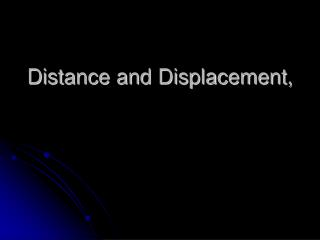# Distance and Displacement, - PowerPoint PPT PresentationDownload PresentationDistance and Displacement,

Distance and Displacement,Download Presentation## Distance and Displacement,

- - - - - - - - - - - - - - - - - - - - - - - - - - - E N D - - - - - - - - - - - - - - - - - - - - - - - - - - -
##### Presentation Transcript

1. Distance and Displacement,

2. Questions for Consideration • What is frame of reference? • What is distance? • How is displacement different from distance?

3. Frame of Reference • Coordinate frame within which to measure position, motion, or other properties of an object. • OR... • Observational frame tied to the motion of an observer. • In Newtonian physics, all motion must be defined in terms of a reference frame. • Relative Motion

4. Reference Frame • Is the speed of the ball different relative to the pitcher, the truck driver, and the jet pilot? Why or why not?

5. 0 1 2 3 4 5 6 7 8 9 10 cm Distance • Distance (d) – how far an object actually travels after leaving its POINT of ORIGIN. • Does not depend on direction. • Imagine an ant crawling along a ruler. • What distance did the ant travel? • d = 3 cm

6. 0 1 2 3 4 5 6 7 8 9 10 cm Distance • Distance does not depend on direction. • Here’s our intrepid ant explorer again. • Now what distance did the ant travel? • d = 3 cm • Does his direction change the answer?

7. 0 1 2 3 4 5 6 7 8 9 10 cm Distance • Distance does not depend on direction. • Let’s follow the ant again. • What distance did the ant walk this time? • d = 7 cm

8. Displacement • Displacement (x) – difference between an object’s final position and its starting position or POINT of ORIGIN. • Does depend on direction. • Displacement = final position – initial position • x = xfinal – xinitial • In order to define displacement, we need directions. • Examples of directions: • + and – • N, S, E, W • Angles

9. Displacement vs. Distance • Example of distance: • The ant walked 3 cm. • Example of displacement: • The ant walked 3 cm EAST. • An object’s distance traveled and its displacement aren’t always the same!

10. - + 0 1 2 3 4 5 6 7 8 9 10 cm Displacement • Let’s revisit our ant, and this time we’ll find his displacement. • Distance: 3 cm • Displacement: +3 cm • The positive gives the ant a direction!

11. - + 0 1 2 3 4 5 6 7 8 9 10 cm Displacement • Find the ant’s displacement again. • Remember, displacement has direction! • Distance: 3 cm • Displacement: -3 cm

12. - + 0 1 2 3 4 5 6 7 8 9 10 cm Displacement • Find the distance and displacement of the ant. • Distance: 7 cm • Displacement: +3 cm

13. Displacement vs. Distance • An athlete runs around a track that is 100 meters long three times, then stops. • What is the athlete’s distance and displacement? • Distance = 300 m • Displacement = 0 m • Why?

14. Dis-City • Create a rectangular city with four roads. • Opposite streets should be the same length(km) • Create at least eight buildings with names. • Add distances between each building spaced around your road. • Add direction • Create 2 questions per person. • Each should have answers for distance and displacemen.t • At least one question should have Pythagorean theorem.

15. Switch Cities and questions with another group and solve. • Groups come together and review questions. • Any questions for the quiz.

16. Scalar and Vector Quantities • Scalar Quantity – has magnitude but not direction. • Distance and speed are scalar quantities. • Vector Quantity – has magnitude and direction. • Displacement and velocity are vector quantities.

17. Speed and Velocity

18. Essential Question • What is speed? • What is velocity? • What are scalar and vector quantities?

19. Speed • Speed (s) – Rate at which an object is moving. • speed = distance / time • s = d/t • Like distance, speed does not depend on direction.

20. 100 m Speed • A car drives 100 meters in 5 seconds. • What is the car’s average speed? • s = d/t • s = (100 m) / (5 s) = 20 m/s 1 s 2 s 3 s 4 s 5 s

21. Speed • A rocket is traveling at 10 km/s. How long does it take the rocket to travel 30 km?

22. Speed • A racecar is traveling at 85.0 m/s. How far does the car travel in 30.0 s?

23. Speed • Since the negative implies direction and speed does not assign direction, speed can only be positive or zero.

24. Velocity • Velocity (v) = speed with direction. • velocity = displacement / time • v = d / t

25. - + 0 1 2 3 4 5 6 7 8 9 10 cm Pulling It All Together • Back to our ant explorer! • Distance traveled: 7 cm • Displacement: +3 cm • Average speed: (7 cm) / (5 s) = 1.4 cm/s • Average velocity: (+3 cm) / (5 s) = +0.6 cm/s 1 s 2 s 3 s 4 s 5 s

26. Graphing

27. Another Look